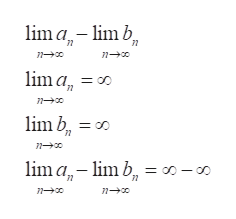# a) Why doesn’t the idea of “any number minus itself is 0” apply to the situation of ∞ − ∞?(b) Explain what ∞ − ∞ means in the context of limits. (c) Assuming the limit exists, what could the result of an ∞ − ∞ limit be and how do you know?

Question

a) Why doesn’t the idea of “any number minus itself is 0” apply to the situation of ∞ − ∞?

(b) Explain what ∞ − ∞ means in the context of limits.

(c) Assuming the limit exists, what could the result of an ∞ − ∞ limit be and how do you know?

check_circleExpert Solution
Step 1

Refer to the questions , we need to give explanation to the provided questions of (a), (b) and (c).

Step 2

(a)…. First of all, infinity is not a number so the value infinity – infinity depends on how we are approaching infinity . So if the term before minus approaches infinity faster, then the result will be infinity and if the it approaches slowly then result is – infinity . Also if they approaches at same rate then the result may be any real number.

So the idea of “any number minus itself is 0” apply to the situation of ∞ − ∞.

Step 3

(b)…. Infinity – infinity in the context ...help_outlineImage Transcriptioncloselima limb lim a = oo lim b, lm a-m b lim b = o0-o D-s n n fullscreen

### Want to see the full answer?

See Solution

#### Want to see this answer and more?

Solutions are written by subject experts who are available 24/7. Questions are typically answered within 1 hour*

See Solution
*Response times may vary by subject and question
Tagged in

### Calculus